﻿﻿ Inductor’s Iron Lossexamples｜products｜Murata Software Co., Ltd.# Example29Inductor’s Iron Loss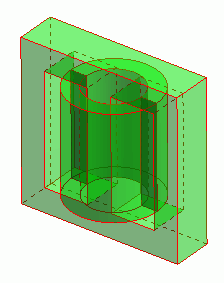General

• A coil is wound on a core. The iron loss is analyzed.

• The core loss, the vectors of the magnetic field and the magnetic flux density are solved.

• Unless specified in the list below, the default conditions will be applied.

• See Exercise 3 regarding the calculation of heating due to the iron loss.

• In this exercise, the permeability of core material is constant (linear). The effect of magnetic saturation is not taken into account.
Refer to [Exercise 8: Iron Loss of Coil (AC Analysis with B-H Curve Taken into Account)] for the analysis with the magnetic saturation taken into account.

### Analysis Space

 Item Settings Analysis Space 3D Model unit mm

### Analysis Conditions

 Item Settings Solvers Magnetic Field Analysis [Gauss] Analysis Type Harmonic analysis Options N/A

The frequency of the current is set to 50[kHz].

 Tab Setting Item Settings Mesh Frequency-Dependent Meshing Reference frequency: 50×10^3[Hz] Surface Treatment for Conductors: Generate skin meshes Harmonic analysis Frequency Sweep Type: Single frequency Frequency: 50×10^3[Hz]

### Model

A coil is wound on a core.

The model is defined by the core (Core) and the loop coil (Coil) are defined.

The loss characteristics are defined in the iron loss table.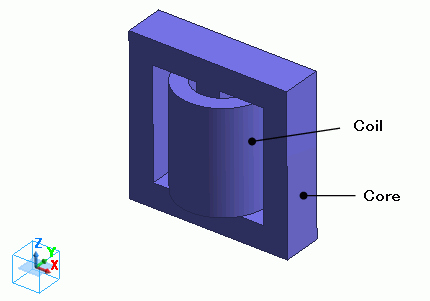### Body Attributes and Materials

 Body Number/Type Body Attribute Name Material Name 5/Solid Coil 008_Cu * 6/Solid Core Core

* Available from the Material DB

The material properties of the core are set up as follows. The loss is defined in the iron loss table.

Material Name

Tab

Settings

Core

Permeability

Relative Permeability: 3000

Conductor wall

Conductivity Type: Conductor

Conductivity: 0.1[S/m]

Iron loss

Iron Loss Calculation Type: Iron loss table

Frequency: 5×10^4[Hz]

[Magnetic flux density-Loss density] Table *

 Magnetic Flux Density [T] Joule Loss Density [W/m^3] 50×10^-3 5×10^3 500×10^-3 500×10^3

* This is not the actual material’s property.

Press the Graph button. The following graph will show up.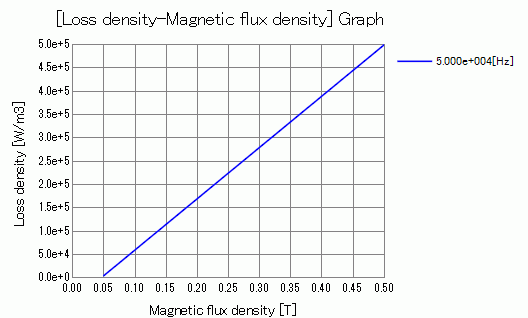Body attribute is set up as follows to apply current to the loop coil.

 Body Attribute Name Tab Settings Coil Current Waveform: AC Current: 0.1[A] Turns: 100[Turns] Direction: Loop Coil/Magnetic Field Direction Direction Vector of Magnetic Field: X=0, Y=0, Z=1

No setting.

### Results

To see the iron loss, go to the [Results] tabclick [Table]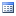.

The joule loss is shown below.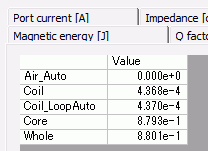The iron loss is shown below.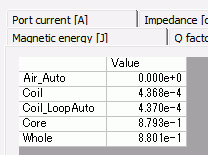The core’s iron loss is approximately 0.9[W].

The joule loss and the hysteresis loss of the core are not given because the the material property is defined on the iron loss table.

The loss characteristics are defined in the iron loss table. Only iron loss is obtained.

If it is defined by the iron loss experimental formula, the joule loss and the hysteresis loss are output as well.

See [Loss Calculation in the Magnetic Field Analysis] for details of the loss.

The vectors of the magnetic field are shown below.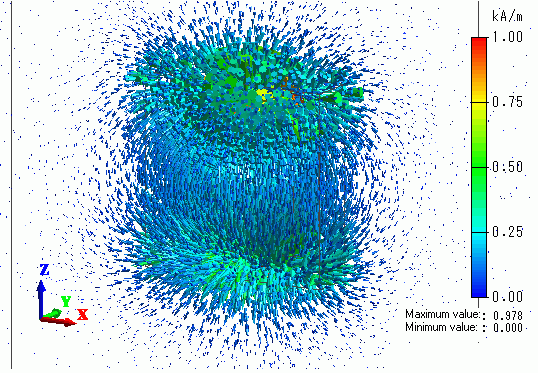The vectors of the magnetic flux density are shown below.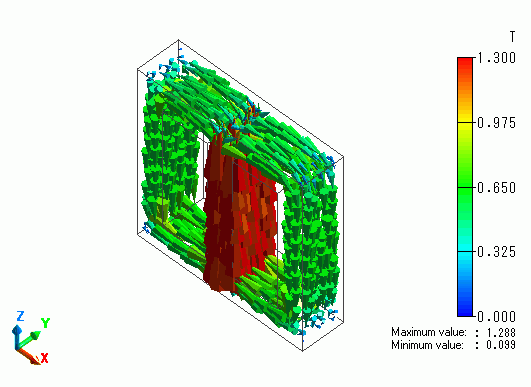The flux density is looping through the core.

The vectors of the current density are shown below.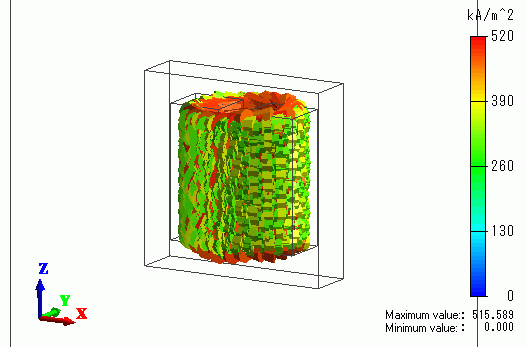The iron loss density is shown below.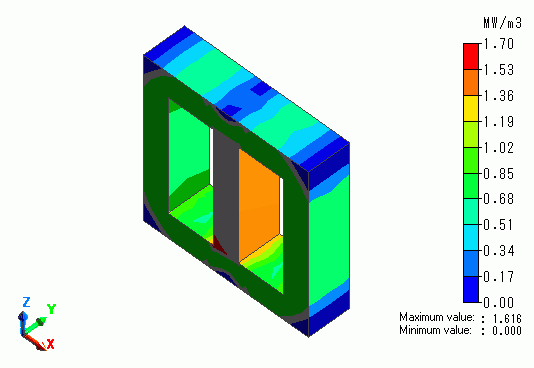﻿ ﻿